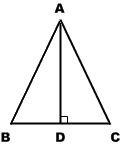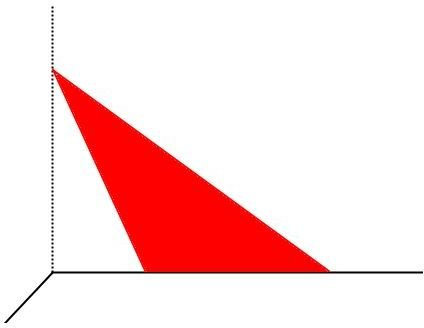# Solve Triangle Area Problems With Pythagorean Theorem

## Area of Isosceles TriangleAn isosceles triangle has two equal sides. If you know the length of all the sides, you can find the area of an isosceles triangle with the help of the Pythagorean theorem. The formula for area of a triangle with base b and height h is

A = ½bh

To find the area of an isosceles triangle ABC, use the unequal side, BC, as the base. The height, AD, intersects the base at the midline and creates two congruent right triangles. The one on the left has hypotenuse AB and leg BD. BD is equal to ½BC. Since the hypotenuse and one leg are known, the Pythagorean theorem can be used to find the length of the third leg, AD, which is also the height of the isosceles triangle.

Example problem: Finding the area of an isosceles triangle

An isosceles has two sides with length 25 cm and a third side with length 30 cm. What is its area?

How to solve:

Step 1. Draw a line segment from the unequal side to the opposite angle. This line is equal to the height and also creates two small right triangles.

Step 2. Find the length of the new line segment using the Pythagorean theorem.

a² + b² = c²

a² + ((½)(30))² = 25² (Why ½ times 30? The short leg is one half of the base of the isosceles triangle.)

a² + 15² = 25² (Remember order of operations. Find the value in parentheses before using exponents outside the parentheses.)

a² + 225 = 625

a² = 400 (Subtract 225 from both sides.)

a = 20 (Find the square root of both sides.)

Step 3. Find the area of the isosceles triangle using the triangle area formula. Use the value of a from Step 2 as the height.

A = ½(30)(20)

A = 300 square inches

## Area of Scalene Triangle

A scalene triangle has three unequal sides. To find its area without knowing its height, you need to know the length of two sides, plus one more value. The height—a perpendicular line segment from the base to the opposite angle—does not divide the base into two equal portions. To calculate the height, you also need the distance along the base from this line segment to one end of the base.

Example problem: Finding the area of a scalene triangleSomeone has painted a mural of a red scalene triangle on a wall (click on the diagram to enlarge it). One corner of the triangle touches the corner between two walls. You decide to paint over it, but you only have a enough white paint to cover 4000 square inches. You already know that the left side is 85 inches long, but you cannot reach high enough to measure the height. So instead, you measure the base (80 inches) and the distance from the base of the triangle to the wall (36 inches). Do you have enough paint?

How to solve:

To solve this problem, look for right triangles so you can use the Pythagorean theorem to find the area.

Step 1. Notice that the red triangle forms a smaller white triangle with the side and bottom of the wall. You know the corner is a right angle, so this is a right triangle. Its base is 36 inches and its hypotenuse is 85 inches.

Step 2. Find the height using the Pythagorean theorem.

a² + b² = c²

a² + 36² = 85² (Cool fact: to square these numbers without a calculator, you can use Vedic mathematics!)

a² + 1296 = 7225

a² = 5929 (Subtract 1296 from both sides.)

a = 77 (Find the square root of both sides—you will need a calculator.)

Step 3. Calculate the area of the red triangle using the area formula for triangles. Use a from Step 2 as the height.

A = ½(77)(80)

A = 3080 square inches

Your 4000 square inches’ worth of white paint will be enough to cover the 3080 square inch red triangle.